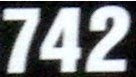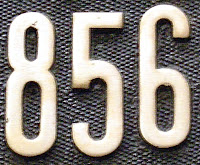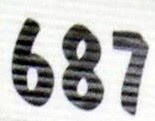## Monday, February 28, 2011

### 149

149 is a prime number.

149 and 151 form a pair of twin primes.

149 has a representation as a sum of two squares: 149 = 72 + 102.

149 is the hypotenuse of a primitive Pythagorean triple: 1492 = 512 + 1402.

149 is the concatenation of the first three positive squares.

149 is the smallest number whose square starts with three identical digits: 1492 = 22201.

149 is the smallest prime that retains the end (unit) digit of its predecessor (139).

149 is the smallest number that is the sum of proper substrings of its reverse: 149 = 1 + 4 + 9 + 94 + 41.149 Medusa is an asteroid discovered in 1875.

## Friday, February 25, 2011

### 351

351 = 33 x 13.

351 is the 26th triangular number. It is the sum of the first 26 numbers. Hence, it is also the numeric value of all the letters of the English alphabet, taken together.

351 is a number that cannot be written as a sum of three squares.

351 is a divisor of 532 - 1.

351 is the smallest number so that it and its immediate neighbors are each the product of four or more primes: 350 = 2 x 5 x 5 x 7; 351 = 3 x 3 x 3 x 13; 352 = 2 x 2 x 2 x 2 x 2 x 11.

The 1971 Ford Mustang Boss 351 was equipped with a powerful 351-cubic-inch V-8 engine.

## Thursday, February 24, 2011

### 874

874 = 2 x 19 x 23.

874 is the number of positive integer solutions to (1 + 1/a)(1 + 1/b)(1 + 1/c)(1 + 1/d)(1 + 1/e) = 2.

874 = 0! + 1! + 2! + 3! + 4! + 5! + 6!

8742 = 763876 = (7 - 6 - 3 + 876)2USS Duncan (DDR 874) was a U.S. Navy destroyer, launched in 1945.

## Wednesday, February 23, 2011

### 742

742 = 2 x 7 x 53.

742 is the maximum number of regions into which 38 lines divide a plane.

742 is the smallest number that is one more than triple its reverse: 742 = 3 x 247 + 1.

742 is the eighth Keith number.GJ 742 (Gliese-Jahreiss 742) is a white dwarf star located about 43 light years from Earth in the constellation Draco.

## Tuesday, February 22, 2011

### 625

625 = 54. It is a perfect fourth power.

Two fourth powers use the same digits: 54 = 625 and 44 = 256.

625 has two representations as a sum of two squares: 625 = 72 + 242 = 152 + 202.

625 is the hypotenuse of a primitive Pythagorean triple: 6252 = 3362 + 5272.According to a 2010 White House news release, 625 people lost their health insurance every hour in 2009.

## Friday, February 18, 2011

### 856

856 = 23 x 107. It is the product of the cube of a prime and a different prime.

856 is 11411 in base 6 and 2332 in base 7.

856 is a member of the Fibonacci-like sequence that begins with 1 and 9: 1, 9, 10, 19, 29, 48, 77, 125, 202, 327, 529, 856, 1385, . . .NWA 856 is a Mars meteorite found in Morocco in 2001.

## Thursday, February 17, 2011

### 714

714 = 2 x 3 x 7 x 17.

The sum of the prime factors of 714 is 2 + 3 + 7 + 17 = 29, and the sum of the prime factors of 715 (5 x 11 x 13) is 5 + 11 + 13 = 29. These numbers are known as Ruth-Aaron pairs.

714 is the smallest number that has equal numbers of every digit in bases 2 and 5: 1011001010 and 10324.

510,510 = 2 x 3 x 5 x 7 x 11 x 13 x 17 (the first seven prime numbers) = 714 x 715 (two consecutive numbers) = 13 x 21 x 34 x 55 (four consecutive Fibonacci numbers).

714 is the sum of twelve consecutive prime numbers: 714 = 37 + 41 + 43 + 47 + 53 + 59 + 61 + 67 + 71 + 73 + 79 + 83.Babe Ruth hit 714 home runs in his baseball career.

## Wednesday, February 16, 2011

### 3208

3208 = 23 x 401.

3208 has a representation as a sum of two squares: 3208 = 382 + 422.

3208 is a divisor of 458 - 1.

3208 is a number that contains exactly three 2s in is base 7 representation: 12232.

3208 is a powerful number because it is the sum of some fixed power of its digits.Caterpillar produces the 3208 diesel engine.

## Tuesday, February 15, 2011

### 316

316 = 22 x 79.

316 is a number that cannot be written as a sum of three squares.

316 has a digit product that is the digit sum of (31)6 = 729: 3 x 1 x 6 = 18; and 7 + 2 + 9 = 18.316 is the telephone area code for the city of Wichita, Kansas.

## Monday, February 14, 2011

### 207

207 = 32 x 23.

207 is a number that cannot be written as a sum of three squares.

207 is the smallest possible sum of primes that are formed using each of the digits 1 through 9: 207 = 89 + 61 + 43 + 7 + 5 + 2.

207 has a fourth power where the digits of the first half are a permutation of the digits of the last half: 2074 = 1836036801.The Peugeot 207 is a supermini automobile first produced in 2006.

## Friday, February 11, 2011

### 805

805 = 5 x 7 x 23.

805 is divisible by the sum of its prime factors.

8052 = 648,025 = (-6 + 4 + 802 + 5)2. The digits of the square of 805 include the digits of 805 as a subsequence.

805 degrees Celsius is 1481 degrees Fahrenheit, a prime temperature.805 is the number of possible positions in the game of checkers after four moves.

## Thursday, February 10, 2011

### 429

429 = 3 x 11 x 13.

429 is the seventh Catalan number.

429 is a divisor of 1003 - 1.

The sum of the digits of 429 is five times the number of digits: 4 + 2 + 9 = 15 = 5 x 3.

The sum of the fourth powers of the factors of 429 is a prime: 34 + 114 + 134 = 43283.The 429 Records label in indie rock/pop and adult alternative music, with a roster that includes Dr. John, Joan Armatrading, and Mick Fleetwood.

## Wednesday, February 9, 2011

### 687

687 = 3 x 229.

687 is a number that cannot be written as a sum of three squares.

687 is 2001 in base 7 and 575 in base 11. It is 22233 in base 4 and 223 in base 18.

687 primes less than 104 do not contain the digit 9.In the Harry Potter books and movies, the Potter family vault number at Gringotts Bank is 687.

## Tuesday, February 8, 2011

### 589

589 = 19 x 31.

589 is the sum of three consecutive primes: 589 = 193 + 197 + 199.

5892 contains exactly six different digits: 5892 = 346921.

589 is the sum of the first 20 semiprimes.The USS Scorpion (SSN-589) was a nuclear submarine of the U.S. Navy.

## Monday, February 7, 2011

### 781

781 = 11 x 71.

781 is a divisor of 55 - 1.

781 is 11111 in base 5.

781 is the maximum number of regions into which 39 lines divide the plane.The oldest recorded eruption of Mt. Fuji in Japan occurred in the year 781.

## Friday, February 4, 2011

### 483

483 = 3 x 7 x 23.

12 x 483 = 5796 and 42 x 138 = 5796. Each equality uses each of the nine digits, 0 to 9, exactly once.

185,472 = 672 x 276 = 384 x 483.

483 is a Smith number because the sum of its digits is equal to the sum of the digits of its prime factors: 4 + 8 + 3 = 3 + 7 + 2 + 3 = 15.WP 483 is a Western Pacific Railroad caboose located at the Western Pacific Railroad Museum (Feather River Railroad Society) in Portola, California.

Source: Numeropedia

## Thursday, February 3, 2011

### 832

832 = 26 x 13.

832 has a representation as a sum of two squares: 832 = 162 + 242.

832 is the maximum number of pieces into which 16 straight slices can cut a torus.

832 is 2266 in base 7; 832 is 31000 in base 4 and 1500 in base 8.832 Karin is the largest member of a cluster of asteroids that formed only 5.8 million years ago.

## Wednesday, February 2, 2011

### 211

211 is a prime number.

211 is the smallest prime such that the sum of its even digits equals the sum of its odd digits.

211 is 111 in base 14.

211 is the maximum number of regions into which 20 lines divide the plane.211 is the number of primes that can appear on a 24-hour digital clock starting from 00:00 up to 23:59.

## Tuesday, February 1, 2011

### 162

162 = 2 x 34.

162 is the only number with a prime factorization of the form n * (n + 1)(nn).

162 has a representation as a sum of two squares: 162 = 92 + 92.

162 is the smallest number with nine representations as a sum of four positive squares: 162 = 12 + 12 + 42 + 122 = 12 + 22 + 62 + 112 = 12 + 42 + 82 + 92 = 12 + 52 + 62 + 102 = 22 + 32 + 72 + 102 = 32 + 42 + 42 + 112 = 32 + 52 + 82 + 82 = 32 + 62 + 62 + 92 = 42 + 42 + 72 + 92.

162 is a divisor of 553 - 1.

162 is an abundant number.

The digit sum of 162 is 9; the digit sum of the square of 162 is 18, and the digit sum of the cube of 162 is 27.162 is the total number of baseball games each team plays during a regular season of Major League Baseball.